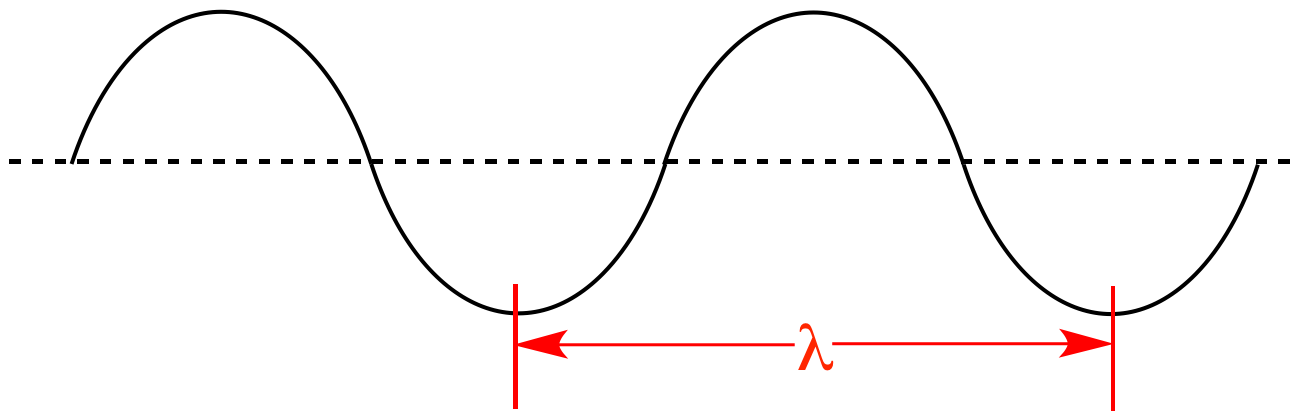Illustrated Glossary of Organic Chemistry

Wavelength (λ): In a wave the distance between any given point and the same point in the next wave cycle. This can be crest-to-crest, trough-to-trough, null-to-null, etc. Wavelength is related to energy and frequency by E = hν = hc/λ, where E = energy, h = Planck's constant, ν = frequency, c = the speed of light, and λ = wavelength.Crest to crest Trough to trough Null to null
Wavelength the distance between any given point and the same point in the next wave cycle.